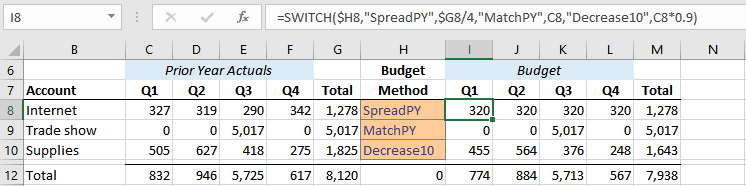## CHOOSE or SWITCH

For decades, Excel users have had the CHOOSE function and it has been able to power some wonderful formulas. But recently, we received the SWITCH function which provides much more flexibility. From a high level, both functions enable us to analyze a value and then return various results based on that value. But, SWITCH offers some options that aren’t available with CHOOSE. So, let’s get to it.

## Objective

Before we get too far, let’s provide some context for these functions. In a previous post, I demonstrated how to allow the user to pick the math with the CHOOSE function. So, we’ll use that as our starting point and then update the workbook with the SWITCH function.

In summary, the previous post showed how to allow the user to enter a budget method code and have the formulas perform the desired math. Here is a screenshot that shows the basic idea along with the formula in cell I17 that uses the CHOOSE function:Depending on what the user enters into the Budget Method column, the Budget formulas will update accordingly. For example, if a user enters code 1, then the budget formulas start with the prior year total and divide by 4 to compute the quarterly amount. If the user enters code 2, the formulas match prior year values. And if the user enters code 3, the formulas decrease the prior year actual by 10%.

Note: here is the full post if you’d like to check it out: Pick the Math with the CHOOSE Function

But, this formula requires the user to enter an integer value of 1, 2, or 3 as the budget method. Let’s say that instead of asking the user to enter a code, we want to let them pick a friendly text label from a drop-down. Well, this is where SWITCH can help.

Note: depending on your version of Excel, you may or may not have the SWITCH function. It is available to O365 subscriptions and Excel 2019 or later.

## SWITCH

The SWITCH function starts by looking at a value. Then, it compares that value with a list of options and returns the corresponding result. If you are familiar with CHOOSE, that sounds about the same. But, there are a few key differences:

• CHOOSE relies on index values (whole numbers 1,2,3…) but SWITCH can use text strings and decimals as well
• CHOOSE requires the options to be listed in order (1, 2, 3…) but SWITCH does not
• CHOOSE will return an error if no matching option is found, but SWITCH allows us to specify a default value that will be returned if no match is found

The syntax for the SWITCH function:

`=SWITCH(expression, value1, result1, value2, result2, ..., default result)`

So, if we wanted to use SWITCH instead of CHOOSE in our budget workbook, it would look like this:The formula basically says look at the budget method value (H8). If it is “SpreadPY” then take the prior year total (G8) and divide by 4. If it is “MatchPY” then just use the prior year amount. If it is “Decrease10” then use 90% of the prior year amount.

This is nice because the user can work with friendly labels like SpreadPY, MatchPY, and Decrease10 instead of integer values such as 1, 2, and 3.

Note: before we had SWITCH, we could use MATCH or VLOOKUP to translate the friendly label to an integer value, and that approach is displayed in the sample file from the previous post.

Plus, we can set up a data validation drop-down so they can pick from a list instead of typing (Data > Data Validation > Allow List).

Also, if we wanted the formula to return a text value of “Unknown” if the user enters a budget method that isn’t in our SWITCH list of options, then we would just add that as the final argument, as follows:

`=SWITCH(\$H8,"SpreadPY",\$G8/4,"MatchPY",C8,"Decrease10",C8*0.9,"Unknown")`

Free free to download the sample file below to check out the formulas for both CHOOSE and SWITCH.

And, if you have any other SWITCH tips, please share by posting a comment below … thanks!

Sample File: ChooseSwitch.xlsx

Posted in ,### Jeff Lenning

I love sharing the things I've learned about Excel, and I built Excel University to help me do that. My motto is: Learn Excel. Work Faster.

### Stay Connected

1.Shimshon on July 3, 2019 at 2:05 pm